## 2  Support classifiers

We begin with the way in which powersets are defined in topos theory, i.e. using the subobject classifier (Section 11) and exponentials, but expressed in a slightly more flexible way. We may arrive at the same definitions from type-theoretic considerations [Tay99, §9.5]. But, whereas the uniqueness of the characteristic map φ is a moot point in that discipline, it is essential to this paper.

The subobject classifier was originally defined by Bill Lawvere in 1969, and the basic theory of elementary toposes was developed in collaboration with Myles Tierney during the following year [Law71, Law00]. Giuseppe Rosolini generalised the definition to classes of supports [Ros86] and developed a theory of partial maps, but the Frobenius law (Propositions 3.11, 8.2 and 10.13) is also required for relational algebra.

Definition 2.1 A class M of morphisms (written ↪) of any category C, such that

1. all isomorphisms are in M,
2. all M-maps are mono, so iM must satisfy ia=iba=b,
3. if i:XY and j:YZ are in M then so is ji, and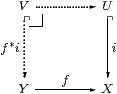4. if i:UX is in M and f:YX is any map in C then the pullback f*i:VY exists in C and belongs to M,

is called a class of supports or a dominion.

Definition 2.2 An M-map ⊤:1→Σ is called a support classifier or a dominance (for M) if for every M-map i:UX there is a unique characteristic map φ:X→Σ making the square a pullback: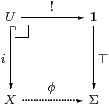Set-theoretically, U is obtained from φ as {x:X ∣ φ[x]} by the axiom of comprehension (separation or subset-selection), though we shall find the abbreviation [φ]↪ X convenient here. Because of the topological intuition we call i:UX (the inclusion of) an open subset. (We shall write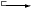for closed subsets and ↣ for Σ-split ones.)

Notice that this pullback is also an equaliser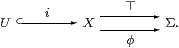The relationship between the axiom of comprehension and the monadic ideas of this paper is explored in Section B 8, which also sets out the λ-calculus that we need in a more explicitly symbolic fashion.

Remark 2.3 This classification property deals only with a single subobject of (or predicate on) X, but in practice we need to consider Γ-indexed families of subobjects, or predicates containing parameters z whose types form the context Γ. These may equivalently be seen as binary relations Γ↽⇀ X or as subobjects of Γ× X, which are classified by maps Γ× X→Σ. We would like these to be given by maps from Γ, i.e. by generalised elements of the internal object of maps X→Σ. Hence we want to use the exponential ΣX. In Set, this is the same as the powerset ℘(X). To summarise, there is a correspondence amongst

i

 z
:U

 z
↪ X,     φ

 z
[x],     φ:Γ× X→Σ    and     ∼ φ: Γ→ΣX≡℘(X)

where ∼ φ(z ) is the subset Uz for (z )∈Γ.

Remark 2.4 We do not intend C to be cartesian closed, because this does not follow from our axioms and we want to use the category of locally compact spaces as an example. Recall that to say that the exponential YX exists in a category C (as a property of these objects individually) means that the functor C(− × X,Y):CopSet is representable, i.e. it is naturally isomorphic to C(−,Z) for some object Z, which we rename ZYX. For this to be meaningful, all binary products Γ× X must first exist in C.

Notice that we ask for exponentials of the form YX for all X and fixed Y, whereas the word exponentiability refers to a property of a particular object X for arbitrary Y. Peter Freyd [FS90] has used the word baseable for the property that we require of Σ, but recognising the meaning of that word out of context depends on already knowing its association with exponents, whereas exponentiating suggests its own meaning more readily. (The word exponent seems to have come from the French exposant [Bar88].)

Remark 2.5 We shall make use of the λ-calculus to define morphisms to and from exponentials such as these. However, since we are only assuming the existence of ΣX, and not cartesian closure, the body of any λ-expression that we use must be of type Σ, or some other provably exponentiating object, such as a retract of ΣY. The range, i.e. the type of the bound variable, is arbitrary (cf. Definition 7.7).

[The restricted λ-calculus that we require, i.e. with just ΣY instead of general exponentials, is sketched in Section A 2.]

We leave it to the reader, making use of some account of λ-calculus and cartesian closed categories such as [Tay99, §4.7], to rewrite juxtapositions like φ(f y) categorically in terms of evaluation (evX× X→Σ), and λ-abstractions as adjoint transpositions. When we write xX, φ∈ΣX etc., we mean generalised elements, i.eC-morphisms x:Γ→ X and φ:Γ→ΣX, or expressions of type X or ΣX involving parameters whose types form an unspecified context Γ; to make Γ explicit in our categorical expressions often involves forming products of various objects with Γ. On the other hand, when we write f:XY, it is sufficient for our purposes to regard this as a particular C-morphism, i.e. a global element of its hom-set, though in most cases parametrisation is possible by reading f as a morphism Γ× XY. At first we need to make the parametrising object explicit (calling it X), as we are considering a new logical principle, but from Section 6 it will slip into the background.

Symbolically, our λ-calculus is peculiar only in that there is a restriction on the applicability of the (→)-formation rule; such a calculus has been set out by Henk Barendregt [Bar92, §5.2], although this is vastly more complicated than we actually need here. We shall instead adopt a much simpler notational convention: lower case Greek letters, capital italics, Σ(−)≡(−)* and the logical connectives and quantifiers denote terms of exponentiating type (predicates), whilst lower case italics denote terms of non-exponentiating type (individuals and functions). The capital italics could be thought of as “generalised quantifiers”.

The objects, unlike the morphisms, are never parametric in this paper (except in Proposition 5.4).

[We also refer to an equation between λ-terms as a statement.]

Remark 2.6 As exponentials are defined by a universal property, the assignment X↦ΣX extends to a contravariant endofunctor, Σ(−):CCop. It takes f:YX to

 Σf:ΣX→ΣY   by   Σf(φ) ≡  φ∘ f ≡  f;φ ≡  λ y.φ(f y).

The effect of Σf is to form the pullback or inverse image along f:Remark 2.7 Returning to the powerset, we write SubM(X) for the collection of isomorphism classes of M-maps into XobC. Then Definition 2.2 says that the contravariant functor SubM:CopSet is representable, i.e. that it is naturally isomorphic to C(− ,Σ) for some object Σ. As a special case of the conditions on M, the pullback (intersection) of any two M-maps into X exists, and the composite across the pullback square is in M, so SubM(X) is a semilattice. In fact, SubM(−) is a presheaf of semilattices on the category C, so C(− ,Σ) is an internal semilattice in the topos of presheaves [Fre66a]. Since the Yoneda embedding is full and faithful and preserves products, Σ was already an internal semilattice in C.

It is well known that this argument makes unnecessary use of the category of sets, which it is the whole point of this paper to avoid (there are also size conditions on C), although we may suppose instead that C is an internal category in some pretopos. However, it is not difficult to disentangle this result from the sheaf theory and prove it directly instead. (The details of this proof would be a useful exercise in diagram-chasing for new students of category theory.)

Proposition 2.8 Any dominance Σ carries a ∧-semilattice structure, and pullback along {⊤}↪Σ induces an external semilattice isomorphism [ ]:C(X,Σ)→SubM(X).Moreover, each ΣX is an internal ∧-semilattice and each Σf is a semilattice homomorphism. (We shall consider the existence and preservation of joins in Section 9.)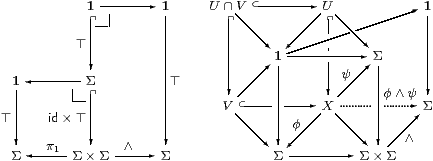Proof    The fifth diagram defines ∧ as sequential and in terms of composition in M; manipulation of the pullbacks in the sixth establishes the relationship with intersection. By construing the monos {<⊤,⊤>}↪Σ×Σ and {<⊤,⊤,⊤>}↪Σ×Σ×Σ as pullbacks in various ways, the semilattice laws follow from the uniqueness of their characteristic maps. These laws are expressed by the four commutative diagrams above involving products, i.e. Σ is an internal semilattice. Moreover, ΣX is also an internal semilattice because the functor (−)X (that is defined for powers of Σ and all maps between them) preserves products and these commutative diagrams. Similarly, Σf is a homomorphism because (−)f is a natural transformation.         ▫

Remark 2.9 In the next section we give a new characterisation of support classifiers as semilattices satisfying a further equation (the Euclidean principle), rather than by means of a class of supports. This characterisation depends on another hypothesis, that the adjunction

 Σ(−)⊣Σ(−)

(which is defined for any exponentiating object Σ) be monadic. The way in which this hypothesis is an abstract form of Stone duality is explored in Examples B 1. In many cases the category C that first comes to mind does not have the monadic property, but [B] constructs the monadic completion C of any category C with an exponentiating object Σ. In fact C is the opposite of the category Alg of Eilenberg–Moore algebras for the monad.

Ultimately our interest is in developing some mathematics according to a new system of axioms, i.e. in the free model (Remark 3.8, Theorem 4.2), but first we introduce the concrete situations on which the intuitions are based. They and Example 4.5 also provide examples and counterexamples to gauge the force of the Euclidean and monadic principles. Even when C is not monadic, its properties are usually close enough to our requirements to throw light on the concepts, without shifting attention to the more complicated C.

[In fact most of the results of this paper can be developed in the similar but alternative framework of equideductive logic, without the monadic principle [DD].]

Examples 2.10

1. Let C be Set or any elementary topos, and Σ≡ Ω its subobject classifier, which is an internal Heyting algebra; classically, it is the two-element set. So ΣX≡ ℘(X) is the powerset of X and M consists of all monos: 1–1 functions or (up to isomorphism) subset inclusions. All objects are compact, overt and discrete in the sense of Sections 68. Section 11 proves Paré’s theorem, that the adjunction Σ(−)⊣Σ(−) is monadic.
2. Let C be any topos and Σ≡ Ωj be defined by some Lawvere–Tierney topology j, so M consists of the j-closed monos [LR75] [Joh77, Chapter 3] [BW85, §6.1]. The full subcategory ℰj of j-sheaves is reflective in ℰ, and the sheaves are the replete objects [BR98]. Restricted to ℰj, the adjunction is monadic, and ℰj is the monadic completion of ℰ.

Examples 2.11

1. Let Σ be the Sierpiński space, which, classically, has one open and one closed point; the open point classifies the class M of open inclusions. Then the lattice of open subsets of any topological space X, itself equipped with the Scott topology, has the universal property of the exponential ΣX so long as we restrict attention to the category C of locally compact sober spaces (LKSp) or locales (LKLoc) and continuous maps [Joh82, §VII 4.7ff]. In this case the topology is a continuous lattice [GHK++80]. A function between such lattices is a morphism in the category, i.e. it is continuous with respect to this (Scott) topology, iff it preserves directed joins [Joh82, Proposition II 1.10].

With excluded middle, all objects are overt, but (unlike Set) LKSp and LKLoc are not cartesian closed, complete or cocomplete. For LKLoc, the adjunction Σ(−)⊣Σ(−) is monadic (§O 5.10 and Theorem B 3.11), as it is for LKSp, assuming the axiom of choice.

2. Let C be the category Cont of continuous lattices and functions that preserve directed joins, and Σ be the Sierpiński space; then ΣX is the lattice of Scott-open subsets of X. In this case Σ(−) is not monadic, but LKLoc is its monadic completion (C) . As Cont is cartesian closed but LKLoc is not, this shows that the construction of C from C in Section B 4 does not preserve cartesian closure. For trivial reasons, all objects of Cont are overt and compact.
3. In these examples we may instead take M to be the class of inclusions of closed subsets, except that now the closed point ⊥ of the Sierpiński space performs the role of ⊤ in Definition 2.2 (Corollary 5.5ff).

It is by regarding (locally compact) frames as finitary algebras over the category of spaces (in which directed joins are “part of the wallpaper”), rather than as infinitary ones over the category of sets, that we achieve complete open–closed duality for the ideas discussed in this paper.

Examples 2.12

1. Let C be Pos and Σ≡Υ≡Ω be the subobject classifier (regarded classically as the poset ⊥⊑⊤), so ΥX is the lattice of upper sets, cf. the notation for the Alexandroff topology in [Joh82, §II 1.8]. The category C is cartesian closed and has equalisers and coequalisers. Although CC is (classically) full and faithful, the real unit interval [0,1] is an algebra that is not the lattice of upper sets of any poset [FW90, Example 9], see also Example B 3.12.
2. The algebras for the monad are completely distributive lattices, but the intuitionistic definition of the latter is itself a research issue [FW90], so we assume excluded middle in our discussion of this example. Nevertheless, Francisco Marmolejo, Robert Rosebrugh and Richard Wood have shown that the opposite of the category of constructively completely distributive lattices is monadic [MRW02]. Classically, this category is equivalent to the category of continuous dcpos and essential Scott-continuous maps, i.e. those for which the inverse image preserves arbitrary meets as well as joins [Joh82, §VII.2]. All objects are overt and compact, whilst Set is embedded as the full subcategory of discrete objects (Example 6.14).

In any given category, there may be many classes M of supports, each class possibly being classified by some object ΣM.

Remark 2.13 Many of the ideas in this paper evolved from synthetic domain theory, a model of which is a topos (with a classifier Ω for all monos) that also has a classifier Σ for recursively enumerable subsets [Ros86, Pho90a, Pho90b, Hyl91, Tay91, FR97, BR98] . In this case, Σ is a subsemilattice of Ω. Such models exist wherein the full subcategory of replete objects satisfies the monadicity property discussed in this paper for Σ [Theorem I 15.10], in addition to that for the whole category for Ω [RT98].